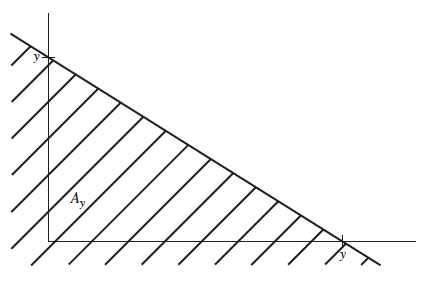Abstract: 本文介绍多随机变量的函数
Keywords: 离散多随机变量的函数，连续多随机变量的函数，卷积

# 多随机变量函数

1. 如果你想进入机器学习这个行业，了解基础技术更重要，你需要会使用python，各种工具包，TensorFlow等基础工具
2. 如果你想在机器学习这个行业稳定的输出而不是撞大运式的调参，你需要了解下网络结构，基础算法，并且你需要非常多的经验去调参。
3. 如果你想成为机器学习的研究者，很遗憾的告诉你，你有一大堆数学要学而且真的不是一两年能学完的，所以还没有毕业的铜须有志于进入研究行列的，大家请多学习数学。

## 有离散联合分布的多随机变量 Random Variables with a Discrete Joint Distribution

Theorem Functions of Discrete Random Variables. Suppose that $n$ random varibales $X_1,\dots ,X_n$ have a discrete joint distribution for which the joint p.f. is $f$ and that $m$ functions $Y_1,\dots ,Y_m$ of these $n$ random variables are defined as follows:
$$Y_1=r_1(X_1,\dots,X_n),\\ Y_2=r_2(X_1,\dots,X_n),\\ \vdots\\ Y_m=r_m(X_1,\dots,X_n)$$
For given values $y_1,\dots,y_m$ fo the $m$ random variables $Y_1,\dots,Y_m$ let $A$ denote the set of all points $(x_1,\dots,x_n)$ such that:
$$r_1(x_1,\dots,x_n)=y_1\\ r_2(x_1,\dots,x_n)=y_2\\ \vdots\\ r_m(x_1,\dots,x_n)=y_m\\$$
Then the value of the joint p.f. $g$ of $Y_1,\dots,Y_m$ is specified at the point $(y_1,\dots,y_m)$ by the relation
$$g(y_1,\dots,y_m)=\sum_{(x_1,\dots,x_n)\in A}f(x_1,\dots,x_n)$$

Theorem Binomial and Bernoulli Distributions. Assume that $X_1,\dots,X_n$ are i.i.d. random variables having the Bernoulli distribution with parameter $p$ .Let $Y=X_1+\dots X_n$ . Then $Y$ has the binomial distribution with parameters $n$ and $p$

1. 可以明确的是，当 $y=x_1+\dots+x_n$ 时，$y$ 的值在 $[0,n]$ 之间
2. 设 $m\in [0,n]$ 那么，根据加法和伯努利分布的性质，其中m个随机变量为1，另外n-m个随机变量为0: $Pr(Y=m)=\begin{pmatrix}n\\m\end{pmatrix}p^{m}(1-p)^{n-m}$
3. 很显然，Y的分布是二项分布。
4. Q.E.D

## 有连续联合分布的多随机变量 Random Variables with a Continuous Joint Distribution

$$\text{for each } y\text{,let }\\ A_y={(x_1,x_2):x_1+x_2\leq y}\\$$$$G(y)=Pr((X_1,X_2)\in A_y)=\int^{y}_{0}\int^{y-x_2}_{0}4e^{-2x_1-2x_2}dx_1dx_2\\ =\int^{y}_{0}2e^{-2x_2}[1-e^{-2(y-x_2)}]dx_2=\int^{y}_{0}[2e^{-2x_2-2e^{-2y}}]dx_2\\ =1-e^{-2y}-2ye^{-2y}$$

Theorem Brute-Force Distribution of a Function.Suppose that the joint p.d.f. of $\vec{X}=(X_1,\dots X_n)$ is $f(\vec{x})$ and that $Y=r(\vec{X})$ For each real number $y$ ,define $A_y={x:r(x)\leq y}$ ,Then the c.d.f. G(y) of Y is:
$$G(y)=\underbrace{\int\dots \int}_{A_y} f(x)dx$$

proof:
$$G(y)=Pr(Y\leq y)=Pr[r(\vec{X})\leq y]=Pr(\vec{X}\in A_y)$$

Theorem Linear Function of Two Random Varibales Let $X_1$ and $X_2$ have joint p.d.f. $f(x_1,x_2)$ and let $Y=a_1X_1+a_2X_2+b$ with $a_1\neq 0$ The $Y$ has a continuous distribution whose p.d.f. is
$$g(y)=\int^{\infty}_{-\infty}f(\frac{y-b-a_2x_2}{a_1},x_2)\frac{1}{|a_1|}dx_2$$

1. 首先我们发现 Y的 c.d.f. G的导数是g，也就是上面定理中的g
2. 对于每一个y，定义 $A_y={(x_1,x_2):a_1x_1+a_2x_2+b\leq y}$
3. $A_y$ 和上面的图（本文就一张图，没错，就是上面例子的那张图） 的情况类似
4. 写出积分限，外部积分到$x_2$ 里层积分是 $x_1$ ,然后就有：
$$G(y)=\int_{A_y}\int f(x_1,x_2)dx_1dx_2=\int^{\infty}_{-\infty}\int^{\frac{(y-b-a_2x_2)}{a_1}}_{-\infty}f(x_1,x_2)dx_1dx_2$$
5. 上面内层积分限有点小复杂，因为y是我们关心的变量，放在内层处理起来会麻烦，所以把他挪到外层。方法就是换元, $z=a_1x_1+a_2x_2+b$ ，那么$x_1=\frac{z-a_2x_2-b}{a_1}$ 那么就有 $dx_1=dz_1/a_1$ 于是内层积分就变成了下面这个式子：
$$\int^{y}_{-\infty}f(\frac{z-b-a_2x_2}{a_1},x_2)\frac{1}{a_1}dz$$
6. 然后我们使用积分的性质做下面的计算：
$$G(y)=\int^{\infty}_{-\infty}\int^{y}_{-\infty}f(\frac{z-b-a_2x_2}{a-1}，x_2)\frac{1}{a_1}dzdx_2\\ =\int^{y}_{-\infty}\int^{\infty}_{-\infty}f(\frac{z-b-a_2x_2}{a_1},x_2)dx_2dz$$

7. 我们可以让内层积分成为一个函数 $t(z)=\int^{\infty}_{-\infty}f(\frac{z-b-a_2x_2}{a_1},x_2)dx_2$ ,然后我们就能得到 $G(y)=\int^{y}_{-\infty}g(z)dz$ 根据微积分基本定理II 其求导等于t(z) ,而 $t{z}$ 就是我们上面定理中的 $g(y)$

8. Q.E.D

Definition Convolution.Let $X_i$ be independent continuous random variables and let $Y=X_1+X_2$ The distribution of $Y$ is called the convolution of the distributions of $X_1$ and $X_2$ .The p.d.f. of $Y$ is sometimes called convolution of the p.d.f.’s of $X_1$ and $X_2$.

$$g(y)=\int^{\infty}_{-\infty}f_1(y-t)f_2(t)dt$$

$$g(y)=\int^{\infty}_{-\infty}f_1(t)f_2(y-t)dt$$

0%